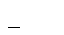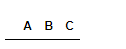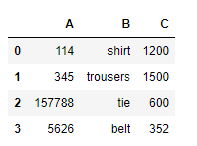Open in App
Not now

# Create empty dataframe in Pandas

• Last Updated : 28 Jul, 2020

The Pandas Dataframe is a structure that has data in the 2D format and labels with it. DataFrames are widely used in data science, machine learning, and other such places. DataFrames are the same as SQL tables or Excel sheets but these are faster in use.
Empty DataFrame could be created with the help of pandas.DataFrame() as shown in below example:

Syntax: pandas.Dataframe()

Return: Return a Dataframe object.

Code:

## Python3

 `# import pandas library``import` `pandas as pd`` ` `# create an empty dataframe``my_df  ``=` `pd.DataFrame()`` ` `# show the dataframe``my_df`

Output:The above output does not show anything lets us insert some heading to the DataFrame.

Code:

## Python3

 `# import pandas library``import` `pandas as pd`` ` `# column name list ``col_names ``=`  `[``'A'``, ``'B'``, ``'C'``]`` ` `# create an empty dataframe``# with columns``my_df  ``=` `pd.DataFrame(columns ``=` `col_names)`` ` `# show the dataframe``my_df`

Output:The empty dataframe showing only headings.

Now let’s Insert some records in a dataframe.
Code:

## Python3

 `# import pandas library``import` `pandas as pd`` ` `# create a dataframe``my_df ``=` `pd.DataFrame({``'A'``: [``'114'``, ``'345'``,``                           ``'157788'``, ``'5626'``], ``                      ``'B'``: [``'shirt'``, ``'trousers'``, ``                           ``'tie'``, ``'belt'``], ``                      ``'C'``: [``1200``, ``1500``, ``                           ``600``, ``352``]}) ``# show the dataframe``my_df`

Output:My Personal Notes arrow_drop_up